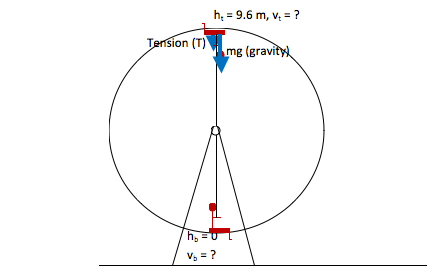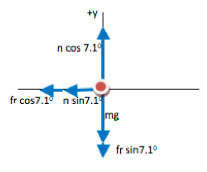# University of Wisconsin Green Bay

### How To Work Physics Problems

There are five key steps to solving any physics problem. Click on any of the links below to see a single step in context of the problem type.

###### How to Identify a ( definition, vector, dynamics, kinematics, energy, momentum, E&M, circuit, induction) problem.How to Draw a Picture for a ( definition, vector, dynamics, kinematics, energy, momentum, E&M, circuit, induction) problem.How to Select the Relation for a ( definition, vector, dynamics, kinematics, energy, momentum, E&M, circuit, induction) problem.How to Solve a ( definition, vector, dynamics, kinematics, energy, momentum, E&M, circuit, induction) problem.How to Understand the Results of a ( definition, vector, dynamics, kinematics, energy, momentum, E&M, circuit, induction) problem.

Scroll down for more detailed information about all five steps, or click the “How To” link under each problem type on the Problems Page to see the full process in context.

### General Approach to Solving Physics Problems

• #### 1. Identify the problem

In order to identify the type of problem that you have, think about the key physics behind your problem. The surface features (in a car, on an incline, on a rope, with or without friction, horizontal or vertical, etc.) do not affect how you solve the problem. Regardless of surface features, all kinematics problems are solved in the same way. Likewise, all dynamics problems are approached in the same way; all energy problems are approached in the same way; etc.

As part of the process of identifying the key physics of the problem, you need to determine what system to consider. In other words, what object (or objects) do you need to track in order to answer the question that you were assigned. The system that you choose for a Conservation of Momentum problem is often very different from the system you would choose for a force problem, and so the questions of key physics and system to consider are inter-linked with each other.

Note that identifying the problem is both the most important and the most frequently skipped step of solving a problem. When you do homework, you generally know the problem type because it is assigned out of a particular chapter of your text. In addition, many books also reference the section number related to each problem. But when it comes time to take your tests, and especially the final exam, you will not have external cues. The few seconds that it takes to clearly articulate to yourself how you know what kind of problem you have (for every homework problem that you work) will truly pay off in both better understanding and better exam scores.

• #### 2. Draw a Picture

Each type of physics problem is paired with a very specific type of picture. The picture that you draw includes all the information that you need to solve the problem (and, ideally, only that information) in a format that pairs directly into the equation that you will use.

Note that once you have identified the problem and drawn the picture, you have put all of your physics understanding into place. All that remains is a mathematical solution for the situation that you have stylized in your picture.• #### 3. Select the Relation

In many cases, once you have identified the key physics of problem that you need to solve, there is only one equation that describes that key physics. In a very few cases (e.g. kinematics,) you may need to select from several relations.

Note that may problems require you to fill in sub-equations as you go along. For example, if you are working with Conservation of Energy, you need to know that gravitational potential energy is given by mgh, or if you work with buoyant force you might need to remember that density is given by m/V. However, you will realize the need for these equations as you come to them. Always begin with the key physics and its corresponding relation.

• #### 4. Solve the ProblemThis step generally gets the most, and deserves the least, attention from students. If you have drawn an appropriate picture, you merely need to put information from the picture into the directly-paired equation and use mathematics that you already know to come up with a numerical solution.

• #### 5. Understand the Results

Once you have completed the problem, look at it again. Does your answer make sense? Did it give the behavior you intuitively expected to find? Can you now do steps that caused you problems earlier? Can you explain in words what is happening? If you only recognized the problem type because of the section heading in your text book, identify the information that you would use to recognize a problem like this on the final exam. A few seconds spent to understand each problem will save you time as you encounter future problems of the same type!